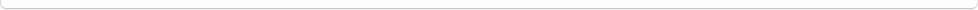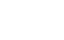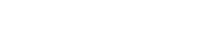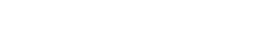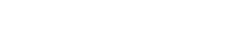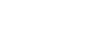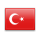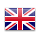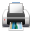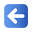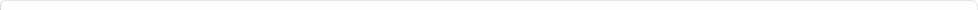Description of Individual Course Units
 Course Unit Code Course Unit Title Type of Course Unit Year of Study Semester Number of ECTS Credits 180102005100 NUMERICAL ANALYSIS Compulsory 3 5 4
Level of Course Unit
First Cycle
Objectives of the Course
To introduce the way of solution techniques of numerical solution of some problems which is difficult or impossible to solve as analytically is aim of this course. On the other hand, the participant (student) would have set a bridge between theoretical subjects have been seen in Scientific and Engineering courses and Mathematical Courses.
Name of Lecturer(s)
Dr. Öğr. Üyesi Züleyha BİNGÜL
Learning Outcomes
 1 Has ability to evaluation of practice problems in engineering and experimental results in laboratory. 2 Has ability to evaluation of practice problems in engineering and experimental results in laboratory. 3 Has ability to get approximate results of difficult problems. 4 Has ability to get approximate results of difficult problems. 5 Has background to make model and solve problems in science and engineering. 6 Has background to make model and solve problems in science and engineering. 7 Control with desired accuracy in numerical solution and results of engineering problems. 8 Control with desired accuracy in numerical solution and results of engineering problems. 9 Derive analytic formulation from experimental results. 10 Derive analytic formulation from experimental results.
Mode of Delivery
Daytime Class
Prerequisites and co-requisities
None
Recommended Optional Programme Components
None
Course Contents
Error Analysis, Solution Methods of Nonlinear Equations, Solution Methods of Linear Set of Equations, Interpolation and Curve Fitting, Numerical Derivation, Numerical Integration, Numerical Solution Methods of Ordinary Differential Equations.
Weekly Detailed Course Contents
 Week Theoretical Practice Laboratory 1 Error Analysis 2 Solution Methods of Nonlinear Equations 3 Solution Methods of Nonlinear Equations 4 Solution Methods of Nonlinear Equations 5 Solution Methods of Nonlinear Equations 6 Interpolation and Curve Fitting 7 Interpolation and Curve Fitting 8 Midterm 9 Numerical Derivation 10 Numerical Derivation 11 Numerical Integration 12 Numerical Integration 13 Numerical Solution Methods of Ordinary Differential Equations 14 Numerical Solution Methods of Ordinary Differential Equations 15 Final
Recommended or Required Reading
Chapra, S.C. ve Canale, R.P., Yazılım ve Programlama Uygulamalarıyla Mühendisler için Sayısal Yöntemler, Türkçesi: Heperkan, H. ve Kesgin, U., Liteatür, İstanbul, 2006. Karagöz, İ., Sayısal Analiz ve Mühendislik Uygulamaları, 3. baskı, Nobel Yayıncılık, 2011. Uzun, İ., Nümerik Analiz, Beta Yayıncılık, 2011. Tapramaz, R., Sayısal Çözümleme, Literatür, İstanbul, 2005. Çengel, Y. A., ve Palm, W. J., (Türçesi: Tahsin Engin), Mühendisler ve Fen Bilimciler İçin Diferansiyel Denklemler, Güven Kitabevi, İzmir, 2012.
Planned Learning Activities and Teaching Methods
Assessment Methods and Criteria
 Term (or Year) Learning Activities Quantity Weight Midterm Examination 1 100 SUM 100 End Of Term (or Year) Learning Activities Quantity Weight Final Examination 1 100 SUM 100 Term (or Year) Learning Activities 40 End Of Term (or Year) Learning Activities 60 SUM 100
Language of Instruction
Turkish
Work Placement(s)
None